# Group 4 2019 September GT TNPSC Question Paper

## Group 4 2019 September GT TNPSC Questions

41.

Express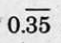into a fraction

A.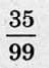B.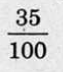C.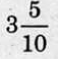D.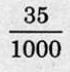A.42.
How many numbers are there between 200 and 300 which are exactly divisible by 6, 8 and 9?
A.
One
B.
Two
C.
Three
D.
Four
B. Two
43.
A can do a piece of work in 20 days and B can do it in 30 days. How long will they take to do the work together?
A.
10 days
B.
12 days
C.
11 days
D.
20 days
B. 12 days
44.
If 14 compositors can compose 70 pages of a book in 5 hours, how many compositors will compose 100 pages of this book in 10 hours?
A.
12
B.
10
C.
8
D.
7
B. 10
45.
Find the simple interest on ₹ 7, 500 at 8% per annum per 1 year 6 months.
A.
₹ 600
B.
₹ 700
C.
₹ 800
D.
₹ 900
D. ₹ 900
46.
Cricket player Dhoni's average in first 30 matches was 72 runs. After 31st match, his average raised as 73 runs. How many runs dis he make in 31st march?
A.
100
B.
103
C.
74
D.
108
B. 103
47.

Arrange in ascending order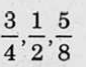A.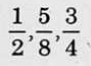B.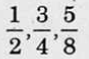C.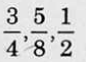D.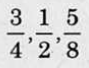A.48.
In a T-20 cricket, Raju hit a "six" 10 times out of 50 balls he played. If a ball was selected at random. Find the probablity that he would not have hit a "six".
A.
1/5
B.
4/5
C.
1/2
D.
3/5
B. 4/5
49.
Right to clean water' is a fundamental right, under the Indian Constitution.
A.
Article 12
B.
Article 21
C.
Article 31
D.
Article 41